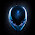## Saturday, 7 May 2011

### Rounding Floating-Point Numbers Up or Down in .NET

#### Introduction

Yesterday, I needed to be able not only to round a floating-point number to a certain number of decimal places, but also control the direction the rounding (i.e.: always force it to round down).

Not a problem, I thought. There will be a method on the `System.Math` class that will let me do just that. How wrong can you be?? My first port of call was the `Math.Round()` method, but whilst you can specify the number of decimal places with this method, you cannot force it to always round either up or down. Next, I looked at `Math.Floor()` and `Math.Ceiling()` methods. These, however, will only round to the nearest integer.

There was nothing else for it, but to roll my own.

#### The Solution

The code for my solution is as follows:

```// C#
public static class EnhancedMath
{
private delegate double RoundingFunction(double value);

private enum RoundingDirection { Up, Down }

public static double RoundUp(double value, int precision)
{
return Round(value, precision, RoundingDirection.Up);
}

public static double RoundDown(double value, int precision)
{
return Round(value, precision, RoundingDirection.Down);
}

private static double Round(double value, int precision, RoundingDirection roundingDirection)
{
RoundingFunction roundingFunction;
if (roundingDirection == RoundingDirection.Up)
roundingFunction = Math.Ceiling;
else
roundingFunction = Math.Floor;
value *= Math.Pow(10, precision);
value = roundingFunction(value);
return value * Math.Pow(10, -1 * precision);
}
}
```
```' Visual Basic
Public Module EnhancedMath

Private Delegate Function RoundingFunction(ByVal value As Double) As Double

Private Enum RoundingDireciton
Up
Down
End Enum

Public Function RoundUp(ByVal value As Double, ByVal precision As Integer) As Double
Return Round(value, precision, RoundingDireciton.Up)
End Function

Public Function RoundDown(ByVal value As Double, ByVal precision As Integer) As Double
Return Round(value, precision, RoundingDireciton.Down)
End Function

Private Function Round(ByVal value As Double, ByVal precision As Integer, ByVal roundingDirection As RoundingDireciton) As Double
Dim roundingFunction As RoundingFunction
If roundingDirection = RoundingDireciton.Up Then
roundingFunction = AddressOf Math.Ceiling
Else
roundingFunction = AddressOf Math.Floor
End If
value = value * Math.Pow(10, precision)
value = roundingFunction(value)
Return value * Math.Pow(10, -1 * precision)
End Function

End Module
```

The rounding algorithm is quite simple: Firstly we shift all the digits we are interested in keeping, to the left of the decimal point. We then either call `Math.Floor()` or `Math.Ceiling()` on the result depending on whether we are rounding down or up respectively. Finally, we shift our digits back to the right of the decimal point and return the value.

The method handles values of type `Double`. You can always add your own overloads to handle values of other types(e.g.: `Decimal`).

#### Some Examples

Here are some examples of our code in action:

```// C#
static void Main(string[] args)
{
Console.WriteLine(EnhancedMath.RoundUp(3.141592654, 2)); // Result: 3.15
Console.WriteLine(EnhancedMath.RoundUp(3.141592654, 3)); // Result: 3.142
Console.WriteLine(EnhancedMath.RoundDown(3.141592654, 2)); // Result: 3.14
Console.WriteLine(EnhancedMath.RoundDown(3.141592654, 3)); // Result: 3.141
Console.Read();
}
```
```' Visual Basic
Sub Main(ByVal args() As String)
Console.WriteLine(EnhancedMath.RoundUp(3.141592654, 2)) ' Result: 3.15
Console.WriteLine(EnhancedMath.RoundUp(3.141592654, 3)) ' Result: 3.142
Console.WriteLine(EnhancedMath.RoundDown(3.141592654, 2)) ' Result: 3.14
Console.WriteLine(EnhancedMath.RoundDown(3.141592654, 3)) ' Result: 3.141
Console.Read()
End Sub
```

#### 2 comments:

1.Doens't work quite well.
1.110m round up to 1.12m.

2.works for me on 0 precision. thanks